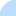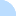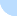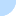## DebcheckDebcheck - jessie  Summary  All packages (light) main only  Broken PreDepends  Broken Depends  Broken Recommends  Broken Suggests  HalfBroken PreDepends  HalfBroken Depends  HalfBroken Recommends  HalfBroken Suggests  Build-Depends  HalfBroken Build-Depends  Malformed Build-Depends  OldLibs  Priority  Old Standards-Version  No Standards-Version  Wrong Standards-Version Syntax other  Depends Main on !Main all sections  Broken PreDepends  Broken Depends  Broken Recommends  Broken Suggests  HalfBroken PreDepends  HalfBroken Depends  HalfBroken Recommends  HalfBroken Suggests  Build-Depends  HalfBroken Build-Depends  Malformed Build-Depends  OldLibs  Priority  Old Standards-Version  No Standards-Version  Wrong Standards-Version SyntaxDistribution: sid bookworm bullseye buster stretch jessie

# Problems for source package nuitka

Current Source Version: 0.5.5.3+ds-1
Current Maintainer: Kay Hayen <kay.hayen@gmail.com>
Section: main
BTS entry: https://bugs.debian.org/src:nuitka

# Half Broken Build-Depends

(not necessarily bugs)

Package declares a build time dependency on base-files (<< 6.1) which cannot be satisfied on armhf. base-files 8+deb8u11 is available. At least one of python3-all-dev (>= 3.2) | base-files (<< 6.1) can be satisfied however.
Package declares a build time dependency on base-files (<< 6.1) which cannot be satisfied on i386. base-files 8+deb8u11 is available. At least one of python3-all-dev (>= 3.2) | base-files (<< 6.1) can be satisfied however.
Package declares a build time dependency on base-files (<< 6.1) which cannot be satisfied on amd64. base-files 8+deb8u11 is available. At least one of python3-all-dev (>= 3.2) | base-files (<< 6.1) can be satisfied however.
Package declares a build time dependency on base-files (<< 6.1) which cannot be satisfied on armel. base-files 8+deb8u11 is available. At least one of python3-all-dev (>= 3.2) | base-files (<< 6.1) can be satisfied however.
Package declares a build time dependency on base-files (<< 6.1) which cannot be satisfied on i386. base-files 8+deb8u11 is available. At least one of python3-all-dbg (>= 3.2) | base-files (<< 6.1) can be satisfied however.
Package declares a build time dependency on base-files (<< 6.1) which cannot be satisfied on armel. base-files 8+deb8u11 is available. At least one of python3-all-dbg (>= 3.2) | base-files (<< 6.1) can be satisfied however.
Package declares a build time dependency on base-files (<< 6.1) which cannot be satisfied on amd64. base-files 8+deb8u11 is available. At least one of python3-all-dbg (>= 3.2) | base-files (<< 6.1) can be satisfied however.
Package declares a build time dependency on base-files (<< 6.1) which cannot be satisfied on armhf. base-files 8+deb8u11 is available. At least one of python3-all-dbg (>= 3.2) | base-files (<< 6.1) can be satisfied however.

# Binary Package: nuitka (Version: 0.5.5.3+ds-1)

BTS entry: https://bugs.debian.org/nuitka

### Half Broken Relationships

(not necessarily bugs)

Package has a Depends on g++-4.4 which cannot be satisfied on armel. At least one of g++-4.9 | g++-4.8 | g++-4.7 | g++-4.6 (>= 4.6.1) | g++-4.5 | g++-4.4 | clang (>= 3.0) can be satisfied however.
Package has a Depends on g++-4.4 which cannot be satisfied on amd64. At least one of g++-4.9 | g++-4.8 | g++-4.7 | g++-4.6 (>= 4.6.1) | g++-4.5 | g++-4.4 | clang (>= 3.0) can be satisfied however.
Package has a Depends on g++-4.4 which cannot be satisfied on i386. At least one of g++-4.9 | g++-4.8 | g++-4.7 | g++-4.6 (>= 4.6.1) | g++-4.5 | g++-4.4 | clang (>= 3.0) can be satisfied however.
Package has a Depends on g++-4.4 which cannot be satisfied on armhf. At least one of g++-4.9 | g++-4.8 | g++-4.7 | g++-4.6 (>= 4.6.1) | g++-4.5 | g++-4.4 | clang (>= 3.0) can be satisfied however.
Package has a Depends on g++-4.7 which cannot be satisfied on i386. At least one of g++-4.9 | g++-4.8 | g++-4.7 | g++-4.6 (>= 4.6.1) | g++-4.5 | g++-4.4 | clang (>= 3.0) can be satisfied however.
Package has a Depends on g++-4.7 which cannot be satisfied on amd64. At least one of g++-4.9 | g++-4.8 | g++-4.7 | g++-4.6 (>= 4.6.1) | g++-4.5 | g++-4.4 | clang (>= 3.0) can be satisfied however.
Package has a Depends on g++-4.7 which cannot be satisfied on armel. At least one of g++-4.9 | g++-4.8 | g++-4.7 | g++-4.6 (>= 4.6.1) | g++-4.5 | g++-4.4 | clang (>= 3.0) can be satisfied however.
Package has a Depends on g++-4.7 which cannot be satisfied on armhf. At least one of g++-4.9 | g++-4.8 | g++-4.7 | g++-4.6 (>= 4.6.1) | g++-4.5 | g++-4.4 | clang (>= 3.0) can be satisfied however.
Package has a Depends on g++-4.5 which cannot be satisfied on armel. At least one of g++-4.9 | g++-4.8 | g++-4.7 | g++-4.6 (>= 4.6.1) | g++-4.5 | g++-4.4 | clang (>= 3.0) can be satisfied however.
Package has a Depends on g++-4.5 which cannot be satisfied on amd64. At least one of g++-4.9 | g++-4.8 | g++-4.7 | g++-4.6 (>= 4.6.1) | g++-4.5 | g++-4.4 | clang (>= 3.0) can be satisfied however.
Package has a Depends on g++-4.5 which cannot be satisfied on i386. At least one of g++-4.9 | g++-4.8 | g++-4.7 | g++-4.6 (>= 4.6.1) | g++-4.5 | g++-4.4 | clang (>= 3.0) can be satisfied however.
Package has a Depends on g++-4.5 which cannot be satisfied on armhf. At least one of g++-4.9 | g++-4.8 | g++-4.7 | g++-4.6 (>= 4.6.1) | g++-4.5 | g++-4.4 | clang (>= 3.0) can be satisfied however.
Package has a Depends on g++-4.6 (>= 4.6.1) which cannot be satisfied on i386. At least one of g++-4.9 | g++-4.8 | g++-4.7 | g++-4.6 (>= 4.6.1) | g++-4.5 | g++-4.4 | clang (>= 3.0) can be satisfied however.
Package has a Depends on g++-4.6 (>= 4.6.1) which cannot be satisfied on amd64. At least one of g++-4.9 | g++-4.8 | g++-4.7 | g++-4.6 (>= 4.6.1) | g++-4.5 | g++-4.4 | clang (>= 3.0) can be satisfied however.
Package has a Depends on g++-4.6 (>= 4.6.1) which cannot be satisfied on armel. At least one of g++-4.9 | g++-4.8 | g++-4.7 | g++-4.6 (>= 4.6.1) | g++-4.5 | g++-4.4 | clang (>= 3.0) can be satisfied however.
Package has a Depends on g++-4.6 (>= 4.6.1) which cannot be satisfied on armhf. At least one of g++-4.9 | g++-4.8 | g++-4.7 | g++-4.6 (>= 4.6.1) | g++-4.5 | g++-4.4 | clang (>= 3.0) can be satisfied however.

Last updated: Thu Oct 28 15:30:09 2021

DO NOT MASS FILE BUG REPORTS
GET A CONSENSUS ON debian-devel@lists.debian.org BEFORE MASS FILING BUGS